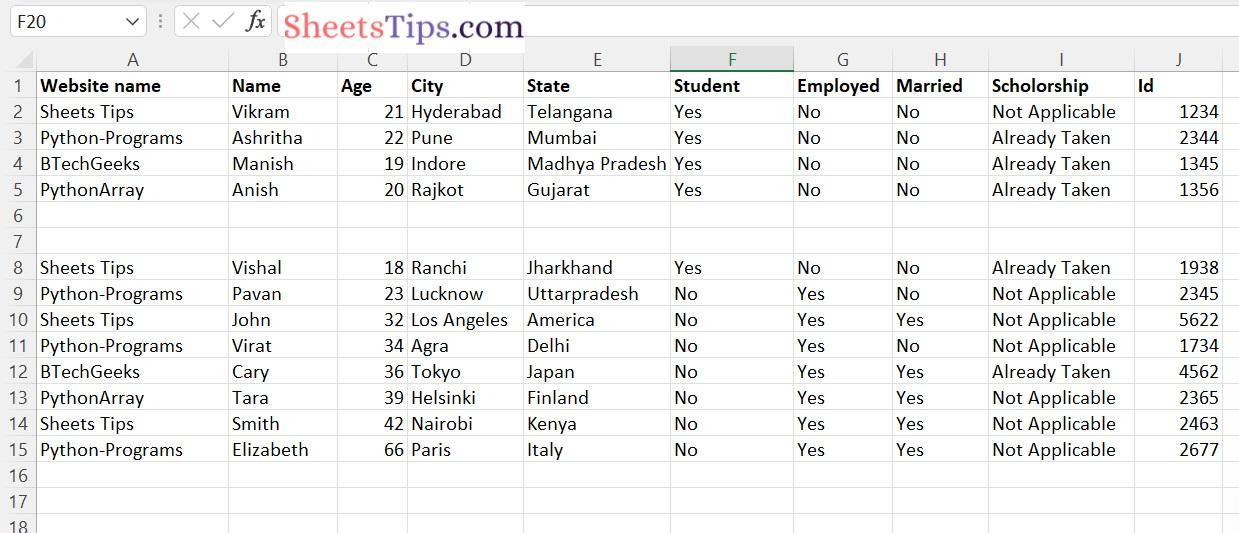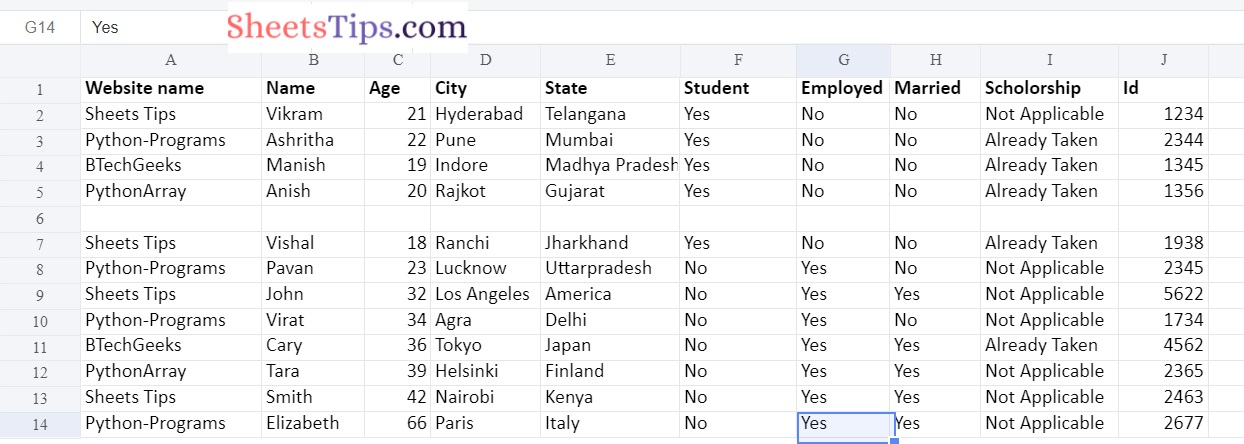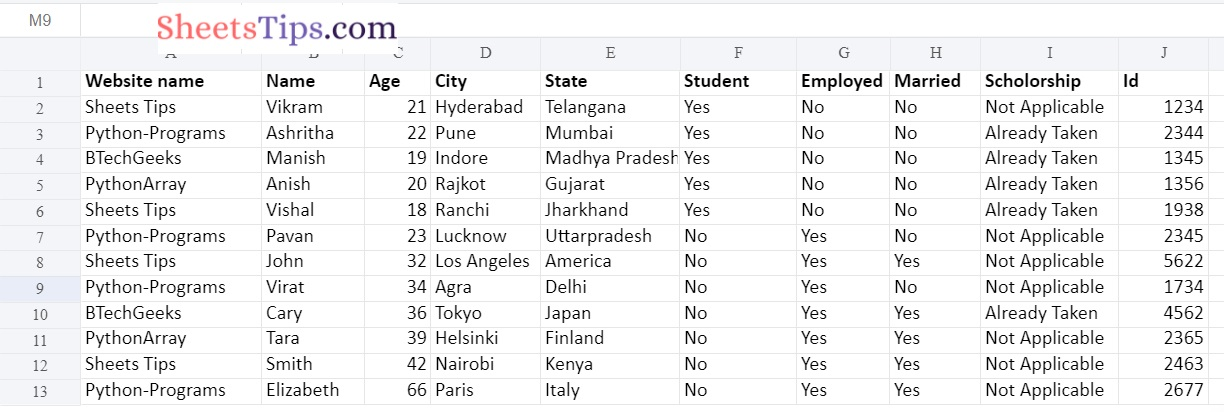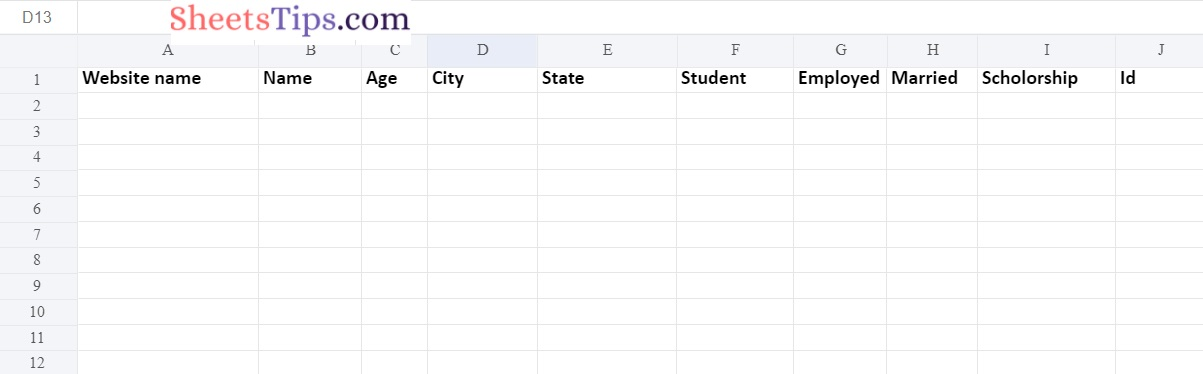# How to Delete One or More Rows in Excel File using Python Openpyxl?

In this tutorial, let us look at how to use openpyxl library to delete rows from an Excel sheet.

Openpyxl Library:

Openpyxl is a Python package that allows you to work with xlsx/xlsm/xltx/xltm files. Openpyxl can be used to create a new Excel file or sheet, as well as to modify an existing Excel file or sheet.

Installation of Openpyxl Library:

The openpyxl is not an in-built module in Python. To install it, run the following command in the terminal.

`pip install openpyxl`

Let us use the sampleExcelFile.xlsx file here which is shown below:

excelFileRowDeletion.xlsx:## Deleting One or More Rows in Excel File using Openpyxl in Python

Below are the ways to delete excel rows:

### Method #1: Delete one/more Empty Rows

1)Removing Empty rows But NOT Continues Empty Rows

In this approach, it removes empty rows but not continuous empty rows, because after the first empty row is deleted, the following row takes its place. As a result, it is not validated.

As a result, recursive function calls can be used to overcome this problem.

Approach:

• Import openpyxl module using the import keyword.
• Create a function say deleteEmptyRows() which accepts excel worksheet and row number as arguments to it.
• Iterate in each cell of the row using the for loop.
• Check the value of each cell in the worksheet row, if any of the values is not None then return.
• It doesn’t remove the row in this case.
• If the row is empty then delete the rows using the delete_rows() function.
• Give the excel file path and store it in a variable.
• Pass the above excel file path as an argument to the load_workbook() function of the openpyxl library to the load the given excel file.
• Choose a particular sheet of the above excel workbook and store it in another variable.
• Print the maximum number of rows in a worksheet before deleting empty rows using the max_row attribute.
• Iterate in the rows of the worksheet object using for loop.
• Pass the worksheet and row number as an argument to the deleteEmptyRows() function.
• Print the maximum number of rows in a worksheet after deleting empty rows using the max_row attribute.
• Give the excel filepath in which the result must be saved and store it in a variable.
• Save the workbook by passing the above filepath as an argument to the save() function.
• The Exit of the Program.

Below is the implementation:

```# Import openpyxl module using the import keyword
import openpyxl

# Create a function say deleteEmptyRows() which accepts excel worksheet, row number as arguments to it.
def deleteEmptyRows(worksheet, row):
# Iterate in each cell of the row using the for loop
for cell in row:
# Check the value of each cell in the worksheet row, if any of the value is not None then return
# It doesnt remove the row in this case
if cell.value != None:
return

# If the row is empty then delete the rows using the delete_rows() function
worksheet.delete_rows(row.row, 1)

# Give the excel file path and store it in a variable
excelFilePath = 'excelFileRowDeletion.xlsx'

# Pass the above excel file path as argument to load_workbook() function of the
# openpyxl library to the load the given excel file

# Choose a particular sheet of the above excel workbook and store it in another variable
worksheet = workbook['Sheet1']
# Print the maximum number of rows in a worksheet before deleting empty
# rows using the max_row attribute
print("The maximum No of rows before deleting empty rows:", worksheet.max_row)

# Iterate in the rows of the worksheet object using for loop
for row in worksheet:
# Pass the worksheet and row number as an arguments to the deleteEmptyRows() function
deleteEmptyRows(worksheet,row)

# Print the maximum number of rows in a worksheet after deleting empty
# rows using the max_row attribute
print("The maximum No of rows after deleting empty rows:",worksheet.max_row)

# Give the excel filepath in which the result must be saved and store it in a variable
newFilePath = 'deleteEmptyRowsExcel.xlsx'
# Save the workbook by passing the above filepath as an argument to the save() function.
workbook.save(newFilePath)```

Output:

```The maximum No of rows before deleting empty rows: 15
The maximum No of rows after deleting empty rows: 14```Explanation:

The first approach just deleted the first empty row, leaving the second continuous empty row unaffected.

2)Removing Empty rows Including Continues Empty Rows

Approach:

• Import openpyxl module using the import keyword.
• Create a function say deleteEmptyRows() which accepts sheet name as an argument to it to remove the empty rows INCLUDING continuous empty rows.
• Iterate through each row of the sheet using the iter_rows() function and for loop.
• Here all() function returns False if all the values of the row are None.
• Remove/delete the empty row in a sheet using the delete_rows() function.
• Call the above deleteEmptyRows() function recursively with the modified sheet data.
• Return it.
• Give the excel file path and store it in a variable.
• Pass the above excel file path as an argument to the load_workbook() function of the openpyxl library to the load the given excel file.
• Choose a particular sheet of the above excel workbook and store it in another variable.
• Print the maximum number of rows in a worksheet before deleting empty rows using the max_row attribute.
• Iterate in each row of the worksheet using the for loop.
• call the above-created deleteEmptyRows() function by passing the worksheet as an argument to it.
• Print the maximum number of rows in a worksheet after deleting empty rows using the max_row attribute.
• Give the excel filepath in which the result must be saved and store it in a variable.
• Save the workbook by passing the above filepath as an argument to the save() function.
• The Exit of the Program.

Below is the implementation:

```# Import openpyxl module using the import keyword
import openpyxl

# Create a function say deleteEmptyRows() which accepts sheetname
# as an argument to it to remove the empty rows INCLUDING continues empty rows.
def deleteEmptyRows(sheet):

# Iterate througn each row of the sheet using the iter_rows() function and for loop
for row in sheet.iter_rows():
# Here all() function returns False if all the values of the row are None
if not all(cell.value for cell in row):

# Remove/delete the empty row in a sheet using the delete_rows() function
sheet.delete_rows(row.row, 1)

# Call the above deleteEmptyRows() function recursively with the modified sheet data
deleteEmptyRows(sheet)
# Return it
return

# Give the excel file path and store it in a variable
excelFilePath  = 'excelFileRowDeletion.xlsx'

# Pass the above excel file path as argument to load_workbook() function of the
# openpyxl library to the load the given excel file

# Choose a particular sheet of the above excel workbook and store it in another variable
worksheet  = workbook['Sheet1']

# Print the maximum number of rows in a worksheet before deleting empty
# rows using the max_row attribute
print("The maximum No of rows before deleting empty rows:", worksheet.max_row)

# Iterate in each row of the worksheet using the for loop
for row in worksheet:
# call the above created deleteEmptyRows() function by passing the worksheet
# as an argument to it
deleteEmptyRows(worksheet)

# Print the maximum number of rows in a worksheet after deleting empty
# rows using the max_row attribute
print("The maximum No of rows after deleting empty rows:",worksheet.max_row)

# Give the excel filepath in which the result must be saved and store it in a variable
newFilePath = 'deleteEmptyRowsContinuous.xlsx'
# Save the workbook by passing the above filepath as an argument to the save() function.
workbook.save(newFilePath)```

Output:

```The maximum No of rows before deleting empty rows: 15
The maximum No of rows after deleting empty rows: 13```### Method #2: Delete All Rows in an Excel File

1)Deleting All Rows

Approach:

• Import openpyxl module using the import keyword.
• Create a function say deleteAllRows() which accepts sheet name as an argument to it to remove the all the rows in an excel file.
• Continue deleting row 2 until there is only one row left with column names using the while loop.
• delete the second row of the sheet using the delete_rows() function.
• Return the result to the main function.
• Give the excel file path and store it in a variable.
• Pass the above excel file path as an argument to the load_workbook() function of the openpyxl library to the load the given excel file.
• select the sheet.
• Print the maximum number of rows in a worksheet before deleting all(except 1st) rows using the max_row attribute.
• Print the maximum number of rows in a worksheet after deleting all(except 1st) rows using the max_row attribute.
• Give the excel filepath in which the result must be saved and store it in a variable.
• Save the workbook by passing the above filepath as an argument to the save() function.
• The Exit of the Program.

Below is the implementation:

```# Import openpyxl module using the import keyword
import openpyxl

# Create a function say deleteAllRows() which accepts sheetname
# as an argument to it to remove the all the rows in an excel file
def deleteAllRows(sheet):
# Continue deleting row 2 until there is only one row left with column names using the while loop
while(sheet.max_row > 1):
# delete the second row of the sheet using the delete_rows() function
sheet.delete_rows(2)
# Return the result to the main function
return

# Give the excel file path and store it in a variable
excelFilePath   = 'excelFileRowDeletion.xlsx'

# Pass the above excel file path as argument to load_workbook() function of the
# openpyxl library to the load the given excel file

# select the sheet
worksheet = workbook['Sheet1']
# Print the maximum number of rows in a worksheet before deleting all(except 1st)
# rows using the max_row attribute
print("The maximum No of rows before deleting : ", worksheet.max_row)

deleteAllRows(worksheet)

# Print the maximum number of rows in a worksheet after deleting all(except 1st)
# rows using the max_row attribute
print("The maximum No of rows after deleting : ", worksheet.max_row)

# Give the excel filepath in which the result must be saved and store it in a variable
newFilePath = 'DeleteAllRows.xlsx'
# Save the workbook by passing the above filepath as an argument to the save() function.
workbook.save(newFilePath)```

Output:

```The maximum No of rows before deleting : 15
The maximum No of rows after deleting : 1```2)Deleting All Rows  with single command

Approach:

• Import openpyxl module using the import keyword
• Give the excel file path and store it in a variable
• Pass the above excel file path as argument to load_workbook() function of the openpyxl library to the load the given excel file
• Choose a particular sheet of the above excel workbook and store it in another variable
• Print the maximum number of rows in a worksheet before deleting all rows (except 1st) using the max_row attribute.
• sheet.max row is the maximum number of rows that the sheet can have. The delete row() method removes rows, where the first parameter is the row number and the second parameter is the number of rows to delete from the row number.
• Print the maximum number of rows in a worksheet after deleting all rows (except 1st) using the max_row attribute.
• Give the excel filepath in which the result must be saved and store it in a variable
• Save the workbook by passing the above filepath as an argument to the save() function.
• The Exit of the Program.

Below is the implementation:

```# Import openpyxl module using the import keyword
import openpyxl

# Give the excel file path and store it in a variable
excelFilePath   = 'excelFileRowDeletion.xlsx'

# Pass the above excel file path as argument to load_workbook() function of the
# openpyxl library to the load the given excel file

# Choose a particular sheet of the above excel workbook and store it in another variable
worksheet  = workbook ['Sheet1']

# Print the maximum number of rows in a worksheet before deleting all rows
# (except 1st) using the max_row attribute
print("The maximum No of rows before deleting all rows(except 1st):", worksheet.max_row)

# sheet.max row is the maximum number of rows that the sheet can have. The delete row()
# method removes rows, where the first parameter
# is the row number and the second parameter is the number of rows to delete from the row number.
worksheet.delete_rows(2, worksheet.max_row-1)

# Print the maximum number of rows in a worksheet after deleting all rows
# (except 1st) using the max_row attribute
print("The maximum No of rows after deleting all rows(except 1st):", worksheet.max_row)

# Give the excel filepath in which the result must be saved and store it in a variable
newFilePath = 'deleteAllRows.xlsx'
# Save the workbook by passing the above filepath as an argument to the save() function.
workbook.save(newFilePath)```

Output: